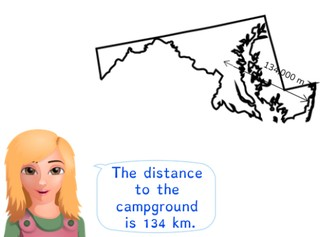Converting m and km

# Converting m and km

The students learn to convert m and km.8,000 schools use Gynzy92,000 teachers use Gynzy1,600,000 students use Gynzy

## General

The students learn to convert meters to kilometers and vice versa.

## Standards

CCSS.Math.Content.4.MD.A.1

## Learning objective

Students will be able to convert meters (m) to kilometers (km) and vice versa.

## Introduction

On the interactive whiteboard there are several statements about meters and kilometers. The students must determine whether each statement is true or false. When they think that the statement is true, they stand up. If they think it is false, they remain seated.

## Instruction

Using a diagram of the metric system, you explain that when you want to convert meters to kilometers, you take three jumps to the left. You also explain that you therefore divide the meters by one thousand, because you take three jumps that are each dividing by ten and 10 × 10 × 10 = 1,000. You then show this by converting the given amounts in meters to kilometers. After this you explain that when you don't have an exact number of kilometers, you have a number of meters left over. You do this by first seeing how many kilometers you have and then seeing how many meters your remainder is. You have the students determine which numbers are missing in the following problems. You also explain that when you want to convert kilometers to meters, you take two jumps to the right and therefore you multiply by one thousand. You show this by converting several lengths in kilometers to meters. Next you explain that when you don't have an exact number of kilometers, you first convert the kilometers to meters and then add the extra meters to that number. The students determine the outcomes of the following problems. Finally you are going to have the students solve story problems. For this, discuss what steps you must follow when solving this type of story problem.

To check whether the students can convert meters to kilometers and vice versa, you can ask the following questions:
- How many meters is one kilometer?
- What do you do when you convert meters to kilometers?
- What do you do when you convert kilometers to meters?

## Quiz

The students test their understanding of converting meters to kilometers and vice versa, through ten exercises. In some of the exercises they must convert measurements in meters to kilometers, and in the others they must convert kilometers to meters. Some of the exercises are story problems.

## Closing

Repeat the learning goal of this lesson and its importance. Next, there are several problems on the interactive whiteboard, for which the students must convert the kilometers to meters and vice versa. You can have the students solve the problems and then check their answers by erasing the covers.

## Teaching tips

When students have difficulty with this learning goal, you can give them a diagram of the metric system, on which there are only meters and kilometers with an arrow for ÷1,000 and an arrow for ×1,000.

## Instruction materials

You may print out a diagram of the metric system.

### The online teaching platform for interactive whiteboards and displays in schools

• Save time building lessons

• Manage the classroom more efficiently

• Increase student engagement